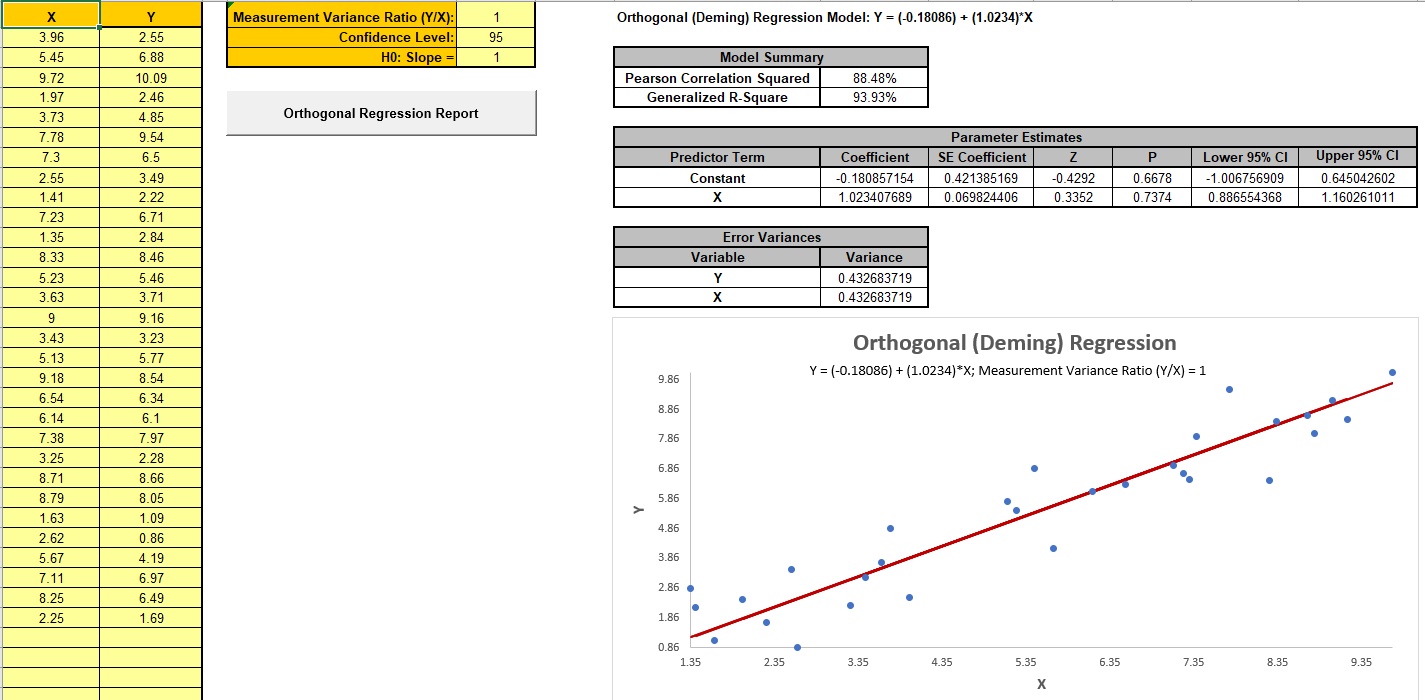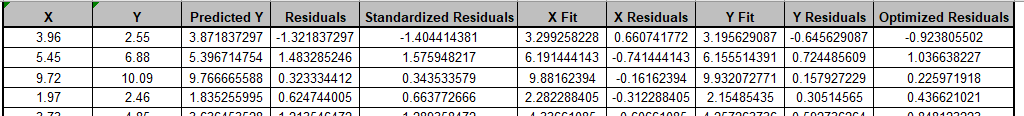Include Top

# Orthogonal (Deming) Regression

Click SigmaXL > Templates & Calculators > Basic MSA Templates > Orthogonal (Deming) Regression to access the Orthogonal (Deming) Regression template.

The following example is given in SigmaXL > Help > Template Examples > MSA > Orthogonal (Deming) Regression.Notes:

1. Orthogonal (Deming) Regression differs from the simple linear regression in that it accounts for errors in observations on both the x- and the y- axis. Use it to compare the same parts on two measurement systems.

2. Enter your Measurement data in the X and Y columns. The X and Y column headings may be modified. This template has a maximum sample size of 1000. Rows with missing data will be deleted.

3. Enter Measurement Variance Ratio (Y/X). A value of 1 assumes equal variance. This ratio is assumed to be constant.

4. The variances for this ratio may be estimated from separate Gage R&R studies or from data replicates. Note that you cannot simply use the variances from the data.

5. Enter Confidence Level and H0: Slope =. Typically H0: Slope = 1, so you are expecting a p-value > alpha, i.e., fail to reject H0.

6. Click the Orthogonal Regression Report button to produce the regression report and scatterplot with orthogonal best fit line.

7. Residuals are given in the report and include Predicted (Raw Y) Residuals, Standardized (Raw Y) Residuals, X Fit Residuals, Y Fit Residuals and Deming Optimized Residuals. See  for formulas.

8. Model Summary includes Pearson Correlation Squared and Generalized R-Square .

9. See SigmaXL Workbook Appendix Orthogonal (Deming) Regression for formula details.

10. SigmaXL must be initialized and appear on the menu in order for this template to function.

REFERENCES

1. Bossé, M., Marland, E., Rhoads, G., Sanqui, J.A. & BeMent, Z. (2022), “Generalizing R2 for deming regressions”, Communications in Statistics - Theory and Methods, DOI: 10.1080/03610926.2022.2059678

2. NCSS Procedures, “Deming Regression”, https://www.ncss.com/wp-content/themes/ncss/pdf/Procedures/NCSS/Deming_Regression.pdf. Note that the error variance ratio is defined as Variance(X)/Variance(Y).

3. Wikipedia “Deming Regression”, https://en.wikipedia.org/wiki/Deming_regression.

ACKNOWLEDGEMENT

Special thanks to the authors of “Generalizing R2 for deming regressions”, Michael Bossé, Eric Marland, Gregory Rhoads, Jose Almer Sanqui & Zack BeMent, for providing R code used to validate Generalized R-Square.

# Web Demos

Our CTO and Co-Founder, John Noguera, regularly hosts free Web Demos featuring SigmaXL and DiscoverSim